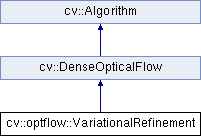OpenCV  3.4.1 Open Source Computer Vision
cv::optflow::VariationalRefinement Class Referenceabstract

Variational optical flow refinement. More...

#include "optflow.hpp"

Inheritance diagram for cv::optflow::VariationalRefinement:## Public Member Functions

virtual void calcUV (InputArray I0, InputArray I1, InputOutputArray flow_u, InputOutputArray flow_v)=0
calc function overload to handle separate horizontal (u) and vertical (v) flow components (to avoid extra splits/merges) More...

virtual float getAlpha () const =0
Weight of the smoothness term. More...

virtual float getDelta () const =0
Weight of the color constancy term. More...

virtual int getFixedPointIterations () const =0
Number of outer (fixed-point) iterations in the minimization procedure. More...

virtual float getGamma () const =0
Weight of the gradient constancy term. More...

virtual float getOmega () const =0
Relaxation factor in SOR. More...

virtual int getSorIterations () const =0
Number of inner successive over-relaxation (SOR) iterations in the minimization procedure to solve the respective linear system. More...

virtual void setAlpha (float val)=0
Weight of the smoothness term. More...

virtual void setDelta (float val)=0
Weight of the color constancy term. More...

virtual void setFixedPointIterations (int val)=0
Number of outer (fixed-point) iterations in the minimization procedure. More...

virtual void setGamma (float val)=0
Weight of the gradient constancy term. More...

virtual void setOmega (float val)=0
Relaxation factor in SOR. More...

virtual void setSorIterations (int val)=0
Number of inner successive over-relaxation (SOR) iterations in the minimization procedure to solve the respective linear system. More...Public Member Functions inherited from cv::DenseOpticalFlow
virtual void calc (InputArray I0, InputArray I1, InputOutputArray flow)=0
Calculates an optical flow. More...

virtual void collectGarbage ()=0
Releases all inner buffers. More...Public Member Functions inherited from cv::Algorithm
Algorithm ()

virtual ~Algorithm ()

virtual void clear ()
Clears the algorithm state. More...

virtual bool empty () const
Returns true if the Algorithm is empty (e.g. in the very beginning or after unsuccessful read. More...

virtual String getDefaultName () const

virtual void read (const FileNode &fn)
Reads algorithm parameters from a file storage. More...

virtual void save (const String &filename) const

virtual void write (FileStorage &fs) const
Stores algorithm parameters in a file storage. More...

void write (const Ptr< FileStorage > &fs, const String &name=String()) const
simplified API for language bindings This is an overloaded member function, provided for convenience. It differs from the above function only in what argument(s) it accepts. More...Static Public Member Functions inherited from cv::Algorithm
template<typename _Tp >
static Ptr< _Tp > load (const String &filename, const String &objname=String())
Loads algorithm from the file. More...

template<typename _Tp >
static Ptr< _Tp > loadFromString (const String &strModel, const String &objname=String())
Loads algorithm from a String. More...

template<typename _Tp >
static Ptr< _Tp > read (const FileNode &fn)
Reads algorithm from the file node. More...Protected Member Functions inherited from cv::Algorithm
void writeFormat (FileStorage &fs) const

## Detailed Description

Variational optical flow refinement.

This class implements variational refinement of the input flow field, i.e. it uses input flow to initialize the minimization of the following functional: $$E(U) = \int_{\Omega} \delta \Psi(E_I) + \gamma \Psi(E_G) + \alpha \Psi(E_S)$$, where $$E_I,E_G,E_S$$ are color constancy, gradient constancy and smoothness terms respectively. $$\Psi(s^2)=\sqrt{s^2+\epsilon^2}$$ is a robust penalizer to limit the influence of outliers. A complete formulation and a description of the minimization procedure can be found in 

## § calcUV()

 virtual void cv::optflow::VariationalRefinement::calcUV ( InputArray I0, InputArray I1, InputOutputArray flow_u, InputOutputArray flow_v )
pure virtual
Python:
flow_u, flow_v=cv.optflow_VariationalRefinement.calcUV(I0, I1, flow_u, flow_v)

calc function overload to handle separate horizontal (u) and vertical (v) flow components (to avoid extra splits/merges)

## § getAlpha()

 virtual float cv::optflow::VariationalRefinement::getAlpha ( ) const
pure virtual
Python:
retval=cv.optflow_VariationalRefinement.getAlpha()

Weight of the smoothness term.

setAlpha

## § getDelta()

 virtual float cv::optflow::VariationalRefinement::getDelta ( ) const
pure virtual
Python:
retval=cv.optflow_VariationalRefinement.getDelta()

Weight of the color constancy term.

setDelta

## § getFixedPointIterations()

 virtual int cv::optflow::VariationalRefinement::getFixedPointIterations ( ) const
pure virtual
Python:
retval=cv.optflow_VariationalRefinement.getFixedPointIterations()

Number of outer (fixed-point) iterations in the minimization procedure.

setFixedPointIterations

## § getGamma()

 virtual float cv::optflow::VariationalRefinement::getGamma ( ) const
pure virtual
Python:
retval=cv.optflow_VariationalRefinement.getGamma()

Weight of the gradient constancy term.

setGamma

## § getOmega()

 virtual float cv::optflow::VariationalRefinement::getOmega ( ) const
pure virtual
Python:
retval=cv.optflow_VariationalRefinement.getOmega()

Relaxation factor in SOR.

setOmega

## § getSorIterations()

 virtual int cv::optflow::VariationalRefinement::getSorIterations ( ) const
pure virtual
Python:
retval=cv.optflow_VariationalRefinement.getSorIterations()

Number of inner successive over-relaxation (SOR) iterations in the minimization procedure to solve the respective linear system.

setSorIterations

## § setAlpha()

 virtual void cv::optflow::VariationalRefinement::setAlpha ( float val )
pure virtual
Python:
None=cv.optflow_VariationalRefinement.setAlpha(val)

Weight of the smoothness term.

getAlpha

## § setDelta()

 virtual void cv::optflow::VariationalRefinement::setDelta ( float val )
pure virtual
Python:
None=cv.optflow_VariationalRefinement.setDelta(val)

Weight of the color constancy term.

getDelta

## § setFixedPointIterations()

 virtual void cv::optflow::VariationalRefinement::setFixedPointIterations ( int val )
pure virtual
Python:
None=cv.optflow_VariationalRefinement.setFixedPointIterations(val)

Number of outer (fixed-point) iterations in the minimization procedure.

getFixedPointIterations

## § setGamma()

 virtual void cv::optflow::VariationalRefinement::setGamma ( float val )
pure virtual
Python:
None=cv.optflow_VariationalRefinement.setGamma(val)

Weight of the gradient constancy term.

getGamma

## § setOmega()

 virtual void cv::optflow::VariationalRefinement::setOmega ( float val )
pure virtual
Python:
None=cv.optflow_VariationalRefinement.setOmega(val)

Relaxation factor in SOR.

getOmega

## § setSorIterations()

 virtual void cv::optflow::VariationalRefinement::setSorIterations ( int val )
pure virtual
Python:
None=cv.optflow_VariationalRefinement.setSorIterations(val)

Number of inner successive over-relaxation (SOR) iterations in the minimization procedure to solve the respective linear system.